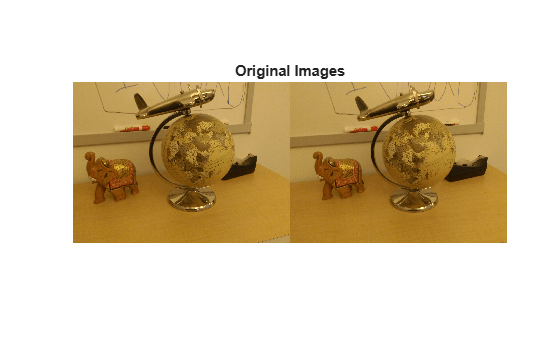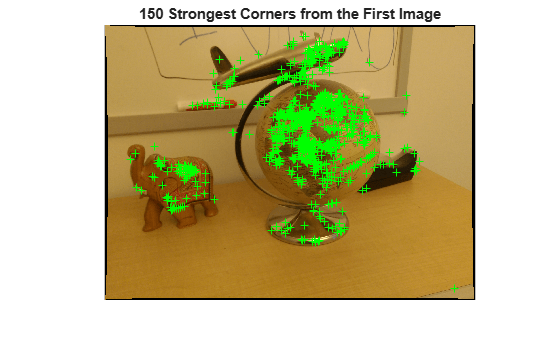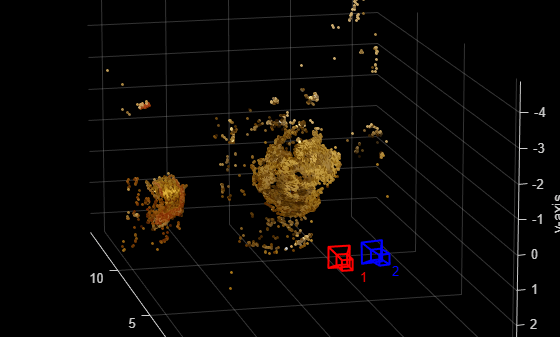# Structure from Motion from Two Views

Structure from motion (SfM) is the process of estimating the 3-D structure of a scene from a set of 2-D images. This example shows you how to estimate the poses of a calibrated camera from two images, reconstruct the 3-D structure of the scene up to an unknown scale factor, and then recover the actual scale factor by detecting an object of a known size.

### Overview

This example shows how to reconstruct a 3-D scene from a pair of 2-D images taken with a camera calibrated using the Camera Calibrator app. The algorithm consists of the following steps:

1. Match a sparse set of points between the two images. There are multiple ways of finding point correspondences between two images. This example detects corners in the first image using the `detectMinEigenFeatures` function, and tracks them into the second image using `vision.PointTracker`. Alternatively you can use `extractFeatures` followed by `matchFeatures`.

2. Estimate the fundamental matrix using `estimateEssentialMatrix`.

3. Compute the motion of the camera using the `estrelpose` function.

4. Match a dense set of points between the two images. Re-detect the point using `detectMinEigenFeatures` with a reduced `'MinQuality'` to get more points. Then track the dense points into the second image using `vision.PointTracker`.

5. Determine the 3-D locations of the matched points using `triangulate`.

6. Detect an object of a known size. In this scene there is a globe, whose radius is known to be 10cm. Use `pcfitsphere` to find the globe in the point cloud.

7. Recover the actual scale, resulting in a metric reconstruction.

### Read a Pair of Images

Load a pair of images into the workspace.

```imageDir = fullfile(toolboxdir('vision'),'visiondata','upToScaleReconstructionImages'); images = imageDatastore(imageDir); I1 = readimage(images, 1); I2 = readimage(images, 2); figure imshowpair(I1, I2, 'montage'); title('Original Images');```This example uses the camera parameters calculated by the Camera Calibrator app. The parameters are stored in the `cameraIntrinsics` object, and include the camera intrinsics and lens distortion coefficients.

```% Load precomputed camera intrinsics data = load('sfmCameraIntrinsics.mat'); intrinsics = data.intrinsics;```

### Remove Lens Distortion

Lens distortion can affect the accuracy of the final reconstruction. You can remove the distortion from each of the images using the `undistortImage` function. This process straightens the lines that are bent by the radial distortion of the lens.

```I1 = undistortImage(I1, intrinsics); I2 = undistortImage(I2, intrinsics); figure imshowpair(I1, I2, 'montage'); title('Undistorted Images');```### Find Point Correspondences Between the Images

Detect good features to track. Reduce `'MinQuality'` to detect fewer points, which would be more uniformly distributed throughout the image. If the motion of the camera is not very large, then tracking using the KLT algorithm is a good way to establish point correspondences.

```% Detect feature points imagePoints1 = detectMinEigenFeatures(im2gray(I1), MinQuality = 0.1); % Visualize detected points figure imshow(I1, InitialMagnification = 50); title('150 Strongest Corners from the First Image'); hold on plot(selectStrongest(imagePoints1, 150));``````% Create the point tracker tracker = vision.PointTracker(MaxBidirectionalError=1, NumPyramidLevels=5); % Initialize the point tracker imagePoints1 = imagePoints1.Location; initialize(tracker, imagePoints1, I1); % Track the points [imagePoints2, validIdx] = step(tracker, I2); matchedPoints1 = imagePoints1(validIdx, :); matchedPoints2 = imagePoints2(validIdx, :); % Visualize correspondences figure showMatchedFeatures(I1, I2, matchedPoints1, matchedPoints2); title('Tracked Features');```

### Estimate the Essential Matrix

Use the `estimateEssentialMatrix` function to compute the essential matrix and find the inlier points that meet the epipolar constraint.

```% Estimate the fundamental matrix [E, epipolarInliers] = estimateEssentialMatrix(... matchedPoints1, matchedPoints2, intrinsics, Confidence = 99.99); % Find epipolar inliers inlierPoints1 = matchedPoints1(epipolarInliers, :); inlierPoints2 = matchedPoints2(epipolarInliers, :); % Display inlier matches figure showMatchedFeatures(I1, I2, inlierPoints1, inlierPoints2); title('Epipolar Inliers');```

### Compute the Camera Pose

Compute the location and orientation of the second camera relative to the first one. Note that `loc` is a translation unit vector, because translation can only be computed up to scale.

`relPose = estrelpose(E, intrinsics, inlierPoints1, inlierPoints2);`

### Reconstruct the 3-D Locations of Matched Points

Re-detect points in the first image using lower `'MinQuality'` to get more points. Track the new points into the second image. Estimate the 3-D locations corresponding to the matched points using the `triangulate` function, which implements the Direct Linear Transformation (DLT) algorithm . Place the origin at the optical center of the camera corresponding to the first image.

```% Detect dense feature points. Use an ROI to exclude points close to the % image edges. border = 30; roi = [border, border, size(I1, 2)- 2*border, size(I1, 1)- 2*border]; imagePoints1 = detectMinEigenFeatures(im2gray(I1), ROI = roi, ... MinQuality = 0.001); % Create the point tracker tracker = vision.PointTracker(MaxBidirectionalError=1, NumPyramidLevels=5); % Initialize the point tracker imagePoints1 = imagePoints1.Location; initialize(tracker, imagePoints1, I1); % Track the points [imagePoints2, validIdx] = step(tracker, I2); matchedPoints1 = imagePoints1(validIdx, :); matchedPoints2 = imagePoints2(validIdx, :); % Compute the camera matrices for each position of the camera % The first camera is at the origin looking along the Z-axis. Thus, its % transformation is identity. camMatrix1 = cameraProjection(intrinsics, rigidtform3d); camMatrix2 = cameraProjection(intrinsics, pose2extr(relPose)); % Compute the 3-D points points3D = triangulate(matchedPoints1, matchedPoints2, camMatrix1, camMatrix2); % Get the color of each reconstructed point numPixels = size(I1, 1) * size(I1, 2); allColors = reshape(I1, [numPixels, 3]); colorIdx = sub2ind([size(I1, 1), size(I1, 2)], round(matchedPoints1(:,2)), ... round(matchedPoints1(:, 1))); color = allColors(colorIdx, :); % Create the point cloud ptCloud = pointCloud(points3D, 'Color', color);```

### Display the 3-D Point Cloud

Use the `plotCamera` function to visualize the locations and orientations of the camera, and the `pcshow` function to visualize the point cloud.

```% Visualize the camera locations and orientations cameraSize = 0.3; figure plotCamera(Size=cameraSize, Color='r', Label='1', Opacity=0); hold on grid on plotCamera(AbsolutePose=relPose, Size=cameraSize, ... Color='b', Label='2', Opacity=0); % Visualize the point cloud pcshow(ptCloud, VerticalAxis='y', VerticalAxisDir='down', MarkerSize=45); % Rotate and zoom the plot camorbit(0, -30); camzoom(1.5); % Label the axes xlabel('x-axis'); ylabel('y-axis'); zlabel('z-axis') title('Up to Scale Reconstruction of the Scene');```### Fit a Sphere to the Point Cloud to Find the Globe

Find the globe in the point cloud by fitting a sphere to the 3-D points using the `pcfitsphere` function.

```% Detect the globe globe = pcfitsphere(ptCloud, 0.1); % Display the surface of the globe plot(globe); title('Estimated Location and Size of the Globe'); hold off```### Metric Reconstruction of the Scene

The actual radius of the globe is 10cm. You can now determine the coordinates of the 3-D points in centimeters.

```% Determine the scale factor scaleFactor = 10 / globe.Radius; % Scale the point cloud ptCloud = pointCloud(points3D * scaleFactor, Color=color); relPose.Translation = relPose.Translation * scaleFactor; % Visualize the point cloud in centimeters cameraSize = 2; figure plotCamera(Size=cameraSize, Color='r', Label='1', Opacity=0); hold on grid on plotCamera(AbsolutePose=relPose, Size=cameraSize, ... Color='b', Label='2', Opacity=0); % Visualize the point cloud pcshow(ptCloud, VerticalAxis='y', VerticalAxisDir='down', MarkerSize=45); camorbit(0, -30); camzoom(1.5); % Label the axes xlabel('x-axis (cm)'); ylabel('y-axis (cm)'); zlabel('z-axis (cm)') title('Metric Reconstruction of the Scene');```### Summary

This example showed you how to recover camera motion and reconstruct the 3-D structure of a scene from two images taken with a calibrated camera.

### References

 Hartley, Richard, and Andrew Zisserman. Multiple View Geometry in Computer Vision. Second Edition. Cambridge, 2000.